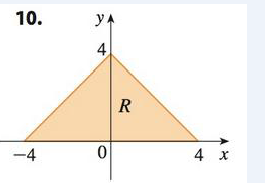Chapter 15, Problem 10RE

Chapter
Section
Textbook Problem

Write ∬ R f ( x ,   y )   d A as an iterated integral, where R is the region shown and f is an arbitrary continuous function on R.To determine

To write: The integral Rf(x,y)dA as an iterated integral.

Explanation

Calculation:

From the given figure, it is observed that the given portion is an triangle. Since, triangular region is given, use of rectangular coordinates is more appropriate to use with. From the given figure, it is observed that the equations of the sides of the triangle are 4y and y4 . So, x varies from 0 to 4 and y varies from y4 to 4y . Thus, the required integral is given by, Rf(x,y)dA=04

Still sussing out bartleby?

Check out a sample textbook solution.

See a sample solution

The Solution to Your Study Problems

Bartleby provides explanations to thousands of textbook problems written by our experts, many with advanced degrees!

Get Started

Find the distance between the points. 5. (2, 5), (4, 7)

Single Variable Calculus: Early Transcendentals, Volume I

In Exercises 1728, use the logarithm identities to obtain the missing quantity.

Finite Mathematics and Applied Calculus (MindTap Course List)

In Exercises 7-28, perform the indicated operations and simplify each expression. 9. 3x2+2x12x+6x21x2+2x3

Applied Calculus for the Managerial, Life, and Social Sciences: A Brief Approach

CHECK POINT True or false: 4.

Mathematical Applications for the Management, Life, and Social Sciences

Solve the equation for x. 44. |3x + 5| = 1

Single Variable Calculus: Early Transcendentals

True or False: is monotonic.

Study Guide for Stewart's Single Variable Calculus: Early Transcendentals, 8th

Draw the garph of y+2=-23x-1.

Elementary Geometry for College Students